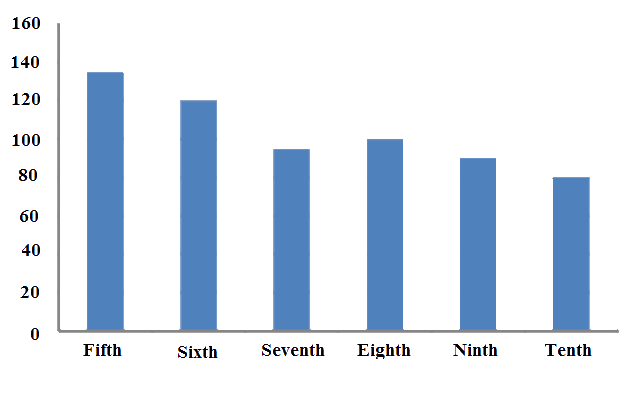# Ex.3.3 Q3 Data Handling - NCERT Maths Class 7

Go back to  'Ex.3.3'

## Question

Number of children in six different classes is given below. Represent the data on a bar graph.

 Class Fifth Sixth Seventh Eighth Ninth Tenth Number of Children $$135$$ $$120$$ $$95$$ $$100$$ $$90$$ $$80$$
1. How would you choose a scale?

(i) Which class has the maximum number of children? And minimum?

(ii) Find the ratio of students of class sixth to the students of class eight?

Video Solution
Data Handling
Ex 3.3 | Question 3

## Text Solution

What is known?

Number of children in different classes.

Reasoning:

We have to draw the graph by using the data.

Steps:a) Scale on y-axis is $$1$$ unit $$= 10$$ children

b)

(i)

• Fifth class has maximum number of children i.e. $$135$$
• Tenth class has the minimum number of children i.e. $$80$$

(ii)

Number of students in class sixth $$=$$ $$120$$

Number of students in class eighth $$=$$ $$100$$

\begin{align} \text{Ratio }&=\frac{\left( \begin{align} & \text{Number of students} \\ & \text{in class sixth} \\ \end{align} \right)}{\left( \begin{align} & \text{Number of students} \\ & \text{in class eighth} \\ \end{align} \right)} \\ & =\frac{120}{100} \\ & =\frac{6}{5} \\ & =6:5 \end{align}

Learn from the best math teachers and top your exams

• Live one on one classroom and doubt clearing
• Practice worksheets in and after class for conceptual clarity
• Personalized curriculum to keep up with school